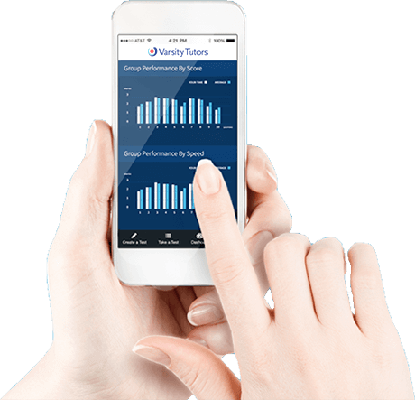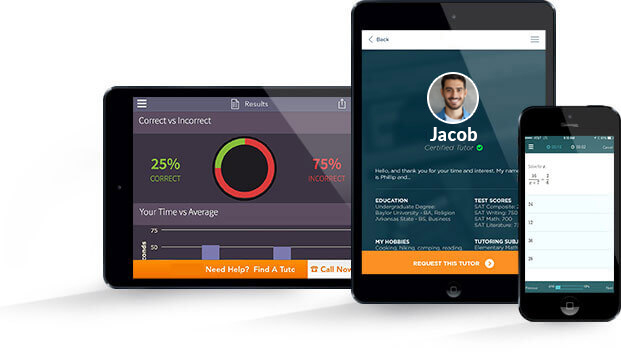# Varsity Tutors Advanced Geometry mobile app### The Varsity Tutors Advanced Geometry Mobile App

Advanced geometry is more comprehensive and covers more complex topics than the basics you have already learned. You’ll learn to graph a logarithm and function. You’ll also learn about cool shapes, like tetrahedrons. Could you find the diagonal of one if asked? Available on iTunes and the Google Play Store, the free Varsity Tutors Advanced Geometry app for Android, iPhone, and iPad provides access to test questions and tools that can deepen your understanding and guide you along toward higher-level math courses.

Advanced geometry involves much more than simply finding area, perimeter, and the volume of shapes. The calculations become more complicated and require more practice to master. You will work with shapes such as rhombuses and trapezoids. You’ll also learn to find the length and area of diagonals. New and different formulas will be introduced and used on problems involving shapes like cones.

The equations and formulas used to solve each problem will need to be carefully chosen and depend on the properties of specific shapes. These are necessary to reach the correct answers for each value. A cone, for example, has unique properties and is three-dimensional. You’ll apply some of the concepts learned in basic geometry, and take those skills to a whole new level. Learning the concepts is not insurmountable. It just takes practice and the right tools to guide you along.

The app is great for daily practice, and can help you as you work toward mastering difficult concepts. All of the questions and content is designed by experts to enhance your understanding of advanced concepts. Use the practice tests to cover as much material as possible or to focus on specific areas. If you want to learn how to graph an ordered pair, for example, you can study only that skill by using the Learn by Concept feature. Full-length practice tests let you work with more general geometry topics.

Mistakes will happen! Use the questions in the practice tests on the app to work through any rough areas during practice. It’s easier to understand the right answer when you can immediately review relevant concept materials. With the Advanced Geometry app, you get exposure to concept-specific materials and can work on solving each problem correctly.If you excel at math subjects, you may choose to take an advanced geometry course. The topics studied in an advanced-level course are basically the same as in other geometry classes, but you are challenged to solve more rigorous and intense problems in your homework and tests. Rather than simply going over the basics of graphing and functions, advanced geometry calls on you to stretch your abilities and really progress deeply into the material, including finding volumes of complex shapes.

One of the most important aspects of advanced geometry is that it combines abstract concepts you have learned in other math courses with the more tangible and visual concept of graphing. There are many problem-solving skills, such as working through multi-step equations and inequalities, you have been taught that will follow through to your geometry class. Have you ever wondered when you would need that algebra you were learning? Well, you definitely need it for your geometry class!

So what exactly is advanced geometry? At the very basic level, coordinate, plane, and solid geometry are covered.

In the first weeks of your course, you will focus on coordinate geometry, in which you will study basic graphing and transformation. Graphing includes how to plot ordered pairs, complex numbers, functions, logarithms, quadratic functions, exponential functions, inverse variations, and two-step inequalities on an x-y axis.

Plotting ordered pairs on a graph is a foundation of geometry. To get acquainted with this, you will first learn how to figure out which quadrant of the graph you will find specific ordered pairs in. The quadrant will depend on whether x and y are positive or negative. There is a specific quadrant for each combination of negative and positive x and y coordinates. From there, plotting points becomes a relatively simple endeavor.

Next, you will get into graphing complex numbers. This can be tricky, in that specific coordinates are often found as a result of calculating imaginary numbers or complex expressions. A number of the concepts you will have to understand are similar to graphing ordered pairs, such as figuring out which quadrant the final answer to the equation will lie in. You will also learn to graph equations using absolute value.

When you move into learning how to graph a function, you will learn to perform tasks such as finding lengths of chords or using the isosceles triangle theorem, the 30-60-90 theorem, and others. You’ll be taught to find the domain of functions, x- and y- coordinates and intercepts of the graphs of functions, and to work with asymptotes.
As function topics build in your course, you will eventually move into graphing logarithms, defining logarithmic functions, and identifying asymptotes for a log function. You’ll also learn about graphing quadratic functions, which result in either a positive (upward facing) or negative (downward facing) parabola, and then you’ll be asked to define certain points on the graph to show you have done it correctly.

Further, when you learn about graphing exponential functions, you will not need to graph many points, as exponentials change only by an exact proportion during a set interval, such as an isotope that decays by half every twenty minutes. To learn this, you’ll be given particular functions (f) and shown how to give the vertical asymptote, and the x- or y-intercept or coordinate, as well as the domain and/or range of functions.

Another important graphing topic in coordinate geometry is inverse variations. This is similar to some of the other graphing concepts, but uses a particular equation, an inverse equation, which is expressed by a formula where the product of two values is equal to a constant.

Finally, you will be graphing two-step inequalities, where you’ll be shown how to do such things as find the equation of a circle given two points that lie on it, choose which line is perpendicular to another line, or find what inequality a given graph represents. You may also learn to find regions on a graph being given certain equations.
The second main topic of coordinate geometry is transformation. Transformation has to do with manipulation of graphs by changing signs. You will learn how to take the shape graphed by an equation, and “flip” it on the graph, coming up with a new equation for the new coordinates.

After you’ve gotten the basics of coordinate geometry covered, the course will move into covering plane geometry. This is where you’ll learn about quadrilaterals, such as squares, rectangles, kites, trapezoids, rhombuses, and other four-sided two-dimensional shapes. The first thing you’ll learn is how to identify each specific quadrilateral, as they each have specific qualities they can be identified by.

In addition to quadrilaterals, you will learn about other 2D shapes, such as circles, hexagons, pentagons, and triangles. You will learn formulas to figure out the area and the length of the diagonal for 2D shapes. You’ll also learn how to find the angle of certain shapes, and their perimeters as well. Specifically, for triangles, you will learn to find out if acute or obtuse isosceles triangles are similar and if they are congruent. Also, you will learn to find the height of a triangle, as well as the length of the hypotenuse of acute and obtuse triangles.

As you move into the more intense topics of your advanced geometry course, you will learn about solid, or 3D, geometry. This involves working with three-dimensional shapes such as cubes, cylinders, prisms, spheres, cones, tetrahedrons, and more. The two most basic things you will learn for each of these shapes is how to find the area of their surface and their volume. This involves, as you may have guessed, adding more formulas to your list. In addition to volume and surface area, you will be learning to find the length of edges on certain shapes (like a prism). For spheres, you will be learning to find the diameter and radius.

In advanced geometry there are many formulas to not only memorize, but to learn how and when to use them. This is often the greatest challenge to geometry students, but it’s a skill you can gain through practice and studying.